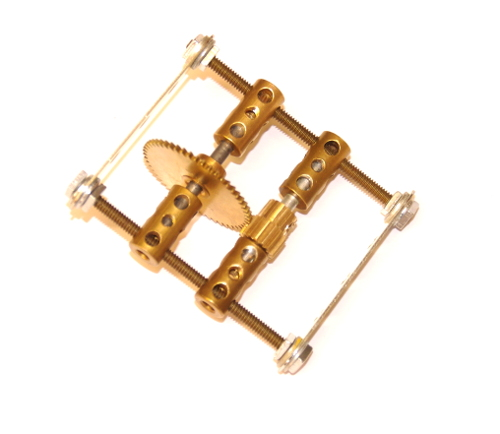Meccano Spares Catalogue

# Meccano Gears - 4 - Meshing at Non-Standard Spacing

## Meccano Gears - 4

### Meshing at Non-Standard Spacing.

The teeth on standard Meccano gears are all cut to the same profile, 38DP (Diametrical Pitch). As all standard Meccano gears are cut to this size, they will all mesh with one another, but not necessarily at factors of ½" pitch.  If you are using gears in a non-Meccano application, and you can machine the centre positions fairly accurately, any pair can mesh together. There is a small amount of simple maths involved, however.

Basically, you need a total of 76 (38 x 2) teeth, for every 1" of pitch. If you remember from previous blog posts, two 38 tooth gears (part 31), mesh together at 1" pitch with a 1:1 ratio. Effectively, any teeth 'removed' from one gear must be 'added' to the other one to keep the centres the same. So, the 3:1 ratio uses 57+19 teeth, which adds up to 76, and the 2:! ratio uses 50+25 teeth, which adds up to 75. (Not 76, I know, but there is a bit of play allowable!)

A worked example. Let's assume you want to mesh the 50 tooth (part 27) and 15 tooth (Part 26d) gears, to give a 3.33:1 ratio. The total number of teeth is 65 (50+15). We need to divide this by the number of teeth at the standard 1" pitch (which is 76), to give the pitch required. 65/76 = 0.855". This can be converted to mm by multiplying by 25.4, so 0.855 x 25.4 = 21.72mm.

Of course, anything is possible with Meccano and a bit of ingenuity....The 3 bore couplings can be positioned at the required spacing along the threaded rods to adapt to other spacings. You can either calculate exactly and measure, using the formula above, or if you're a bit more 'hands on', just twiddle the couplings until the gears mesh nicely!

 Share TweetMeccano Spares
 View Basket | Wish List | New Account | Sign In
 Search for Meccano Parts: